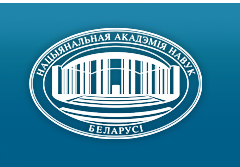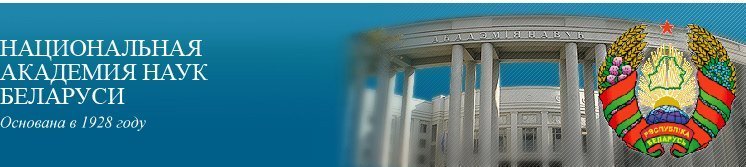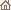Главная Новости Об академии Деятельность академии Члены академии Организации Издания академии Мероприятия Контакты Электронные обращения Справочная информация Карта сайта

Известия Нац. академии наук Беларуси. Сер. физ.-матем. наук, 1998, No.4/ Издания академии / Научные журналы

Известия Нац. академии наук Беларуси. Сер. физ.-матем. наук, 1998, No.4Известия Национальной академии наук Беларуси СЕРИЯ ФИЗИКО-МАТЕМАТИЧЕСКИХ НАУКИздатель: Беларуская навука, Минск, Республика Беларусь

Номер 4, 1998СОДЕРЖАНИЕ

МАТЕМАТИКА

Gaishun I. V., Izobov N. A.
Research on differential and multiparameter systems at the Institute of Mathematics of the National Academy of Sciences of Belarus. pp. 5--19

Summary: The summary contains results in the general theory of dynamic systems, mathematical control theory and the asymptotic theory of linear and nonlinear differential systems obtained in last ten years at departments of differential equations and mathematical theory of systems of the Institute of Mathematics of the National Academy of Sciences of Belarus.

Matus P. P., Sobolevsky P. I., Yanovich L. A.
On some results in the theory of approximate and numerical methods. pp. 20--36

Summary: A brief survey of results in approximate methods of analysis, numerical methods for solving mathematical physics problems, and parallel computing methods obtained at the Institute of Mathematics of the National Academy of Sciences of Belarus is given. Results of the last 20 years are mainly considered.

Gromak V. I., Stepanova T. S.
On general representation of meromorphic solutions for higher analogues of the second Painleve equation. pp. 37--40

Summary: A representation of meromorphic solutions for higher analogues of the second Painleve equation as a ratio of entire functions is offered, A bijective correspondence between the meromorphic solutions of these equations and the entire solutions of systems constructed for them is established.

Gorokhovik V. V., Simonov A. Yu.
Optimality conditions in the terminal control problem for an output of a discrete system with respect to a preference relation. pp. 41--46

Summary: A nonlinear discrete control system with a vector output is considered. Necessary optimality conditions of the first and the second orders are given.

Bernik V. I., Morozova I. M.
Diophantine approximations with the lacunary polynomials and the Hausdorff dimension. pp. 47--51

Summary: We obtained the exact value of the Hausdorff dimension for the set of points in the complex plane at which the lacunary polynomials of the third degree of the form a3 zm + a2 zn + a1 zl + a0 approximate zero with the given error.

Chizh O. P.
Contact geometry of hyperbolic Monge-Ampere equations. pp. 52--56

Summary: We show that an invariant Cartan connection can be associated with every generic hyperbolic Monge--Ampere equation. A local classification of equations with a maximal symmetry group is obtained (up to the group of contact transformations).

ФИЗИКА

Borisevich N. A., Tolkachev V. A., Blohin A. P., Povedailo V. A.
Dynamics of the rotational molecules in the gas phase. pp. 57--64

Summary: The paper reviews of the author's research on the optical manifestation rotational dynamics of polyatomic molecules is given.

Bogush A. A.
To the 40-th anniversary of Fedorov's vector parametrization. pp. 65--72

Summary: The development and applications of the vector parametrization of the classical groups SO(3.R) and SO(3.1) systematically elaborated by F.I.Fedorov 40 years ago, are briefly reviewed and analyzed. The main attractive properties and some advantages of the vector parametrization as the mathematical foundation for developing a general method of finite physical symmetry transformations are indicated. Main results in the relativistic kinematics of elementary particle interactions obtained with the help of finite Lorentz transformations (including their quaternionic form), as well as some basic problems in the gauge field theory solved by using of local vector parameters of the dynamical symmetry transformations are briefly discussed.

Burakov V. S., Raikov S. N.
Plasma diagnostics of an impulsed discharge in xenon by an intracavity laser spectroscopy method. pp. 73--81.

Summary: The intracavity laser spectroscopy is used for the plasma diagnostics of a high-current pulsed discharge in xenon at lowered pressures. The main attention is paid to the dynamics of transparent quartz wall ablation during a discharge pulse and to the influence of evaporated species on parameters of the gas plasma.

Batay L. E., Gribkovskii V. P., Demidovich A. A., Kuzmin A. N., Rybtsev G. I.
Laser diode pumped solid-state sources of coherent radiation. pp. 82--91

Summary: The most perspective ways of developing laser diode pumped solid-state laser systems are analyzed. Characteristics of active media, nonlinear crystals, different pump schemes, as well as the results of original research on the laser diode pumping obtained at the Institutes of Physics of NASB and of Molecular and Atomic Physics of NASB jointly with the State Enterprise "LEMT" are presented.

Makovetskii G. I., Ryzhkovskii V. M., Fedosyuk V. M.
Properties and phase diagrams of some magnetically ordered systems. pp. 92--100

Summary: A survey of the investigations on the structural magnetic, electrical properties and magnetic phase diagrams of ferrites, manganites, orthoferrites, ferrite-garnets, chalcogenides and pnictides of the 3d-transition elements with the NiAs- and Cu2Sb-type structures performed at the Institute of Solid State and Semiconductor Physics of the National Academy of Sciences of Belarus is presented. Results on thin magnetic films and nanoscale structure based on the ferrum group with dia- and paramagnetic metals are outlined.

Sirota N. N., Vitkina Ts. Z.
Electron density distribution for C--C, C--O bond fragments. pp. 101--105

Summary: The electron density distribution for C--C and O--C bonds in dependence of the type of atomic wave function hybridization, atom state and the degree of bond covalency is investigated. It is shown that the strength of the bonds formed by hybridized functions of excited states is small as compared to the characteristics obtained while hybridizing atoms in the stationary state.

Pashkovskii O. I., Olekhnovich N. M., Jarunichev V. P., Kurilovich N. F.
Effects of g-radiation on properties of semiconducting barium titanate -- based ceramics. pp. 106--110.

Summary: Results on electrical properties of a semiconducting posistor ceramics of barium titanat irradiated by g (Co60)-radiation arc given. The radiation effects in the ceramics are found to depend on its composition. The degradation process of the ceramic containing SiO2 precipitation is appeared to be accelerated by the g-radiation.

Zharkevich A. V., Klintsevich S. I., Narkevich I. I.
A statistic-mechanical description of structural, thermodynamic and mechanical properties of a nonuniform molecular medium. pp. 111--117

Summary: An opportunity to develop a statistical theory of a condensed molecular media which can describe equilibrium properties of molecular systems from a united initial position is discussed. In particular, an analytical state equation of a model condensed molecular media is obtained in the framework of a two level statistical description of nonuniform medias. Statistical calculation of contributions to thermodynamic potentials from fluctuating density fields in a configuration space arc made. A nonlinear integral equation for potentials of average forces determining junior correlative functions of the particle distribution in a nonuniform deformed crystal is used. These potentials are expanded in a functional row at the components of the deformation tensor. A system of linear integral equations of Fredholm's type of the second kind is obtained for the expansion coefficients.

Dik S. K.
Investigating the spectrum of a scattered coherent radiation under longitudinal oscillations of a diffuser. pp. 118--120

Summary: Fluctuation spectra of speckle-field tension under longitudinal oscillations of diffusion-passing objects are investigated. Calculation results and experimental data are given. Dependence of the ratio of two the first spectrum harmonics on the oscillation amplitude and the parameters that characterize the lightening beam are determined.

ИНФОРМАТИКА

Levin G. M., Tanaev B. C.
A parameter decomposition of optimization problems. pp. 121--131

Summary: An extended parameter decomposition of optimization problems is considered. The parametrization is used as an uniform basis for complex application of such machinery as decomposition and. immersion of obtained subproblems in more simple problems. The main attention is paid for a) a general decomposition scheme, b) sufficient conditions of its applicability, c) interrelations of so-called stationary and local areas of subproblems obtained and an initial problem, d) some techniques for an extended parameter decomposition of mathematical programming problems.

Chernyavski A. F.. Kolyada A. A., Revinski V. V., Selyaninov M.Yu., Shabinskaya E. V.
Scaling methods for minimal redundant modular arithmetics. pp. 132--137

Summary: A new scaling method in minimal redundant modular number systems is offered. A method developed allows one to lower the volume of hardware expenses by 57% in comparison with a universal scaling method (oriented on any scale) and by 47.7% in comparison with an updating of this method designed for scales of a particular kind, which are products of some modules of the system.

УЧЕНЫЕ БЕЛАРУСИ

Алферов Ж.И., Апанасевич П.А., Астапчик С.А. и др.
Николай Николаевич Сирота (К 85-летию со дня рождения). С. 138--140
Нажмите
здесь для чтения статьи/ Издания академии / Научные журналы / К началу страницы

Разработана и поддерживается Николаем Н. Костюковичем. Последнее обновление: 7 ноября 2006 г.
Создана при участии Игнатия И. Корсака
Копирайт © 1999-2006 Национальная академии наук Беларуси
Копирайт © 1998 Издательство "Беларуская навука"